# 2 – PvT behavior of pure components

### Textbook Examples:

XPS-file display is available in Internet Explorer. In case of Firefox, select Internet Explorer when asked for the software to open the file.

02.06          Isobaric Heating of Ethylene Using a High Precision Equation of State.
(the link to the reference “02.EOS-ethylene.mcd” has to be deleted and added new via Insert – Reference !)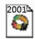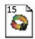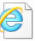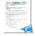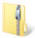02.07           Zeno line, Joule-Thomson Inversion Curve and Boyle Curve Using a High Precision Equation of State.
Missing due to convergence problems that need to be solved.P02.01         Compressibility Factor and Molar Volume of Methanol Steam
Calculate the compressibility factor and the molar volume of methanol steam at 200°C and 10 bar

a) using the ideal gas law
b) with the virial equation truncated after the 3rd   virial coefficient

(virial coefficients: B=-219 cm3/mol; C=-17,300 cm6/mol
2)P02.02        Pressure and Compressibility Factor of Ethylene Using the Virial and the Peng-Robinson Equation of State

A container with a volume of V=0.1 m3 is filled with m = 10 kg ethylene at a temperature of T = 300 K. What will be the
pressure and compressibility factor of the gas? Use the virial equation with only two coefficients and the Peng-Robinson equation of state to describe the PVT-behavior. (virial coefficient: B=-138 cm3/mol, all other properties are given in Appendix A)Mathcad (2001) – Solution as XPS

P02.03        Calculation of the 2nd Virial Coefficient from the VdW EOS

Derive an expression for the 2nd virial coefficient based on the van der Waals equation of state.Mathcad (2001) – Solution as XPS

P02.04        Volume Dependence of the Internal Energy from the VdW EOS

Derive an expression for the volume dependence of the internal energy U at constant temperature based on the van der Waals equation of state.Mathcad (2001) – Solution as XPS

P02.05        Pressure of CO2 from Ideal Gas Law, Virial Equation and Redlich-Kwong EOS

Calculate the pressure of 1 mol CO2 in a container of 2.5 dm3 at 40°C via the
a) ideal gas law
b) virial equation
c) Redlich-Kwong-equation
(virial coefficient: B=-110 cm3/mol; for all other required properties see Appendix A)Mathcad (2001) – Solution as XPS

P02.06        Derivation of Residual Functions Using the Redlich-Kwong EOS

Derive the expressions for the residual functions (h-hid), (s-sid), (g-gid) using the Redlich-Kwong equation of state.Mathcad (2001) – Solution as XPS

P02.07        Change in Enthalpy During Isothermal Compression

Calculate the change in enthalpy of 1 mol of liquid ethanol during isothermal compression from 1 bar to 100 bar at a temperature of 25°C. The compressibility coefficient (1.14·10-4 bar-1), the thermal expansion coefficient (1.1·10-3 K-1) and the molar volume (58.04 cm3/mol) are regarded as constant.Mathcad (2001) – Solution as XPS

P02.08        Virial Coefficient Data Via the DDB

Search for experimental 2nd virial coefficient data for nitrogen in the DDB. Compare the values to the estimation results from the Tsonopoulos method via DDBSP-Predict. Estimate the Boyle temperature from the experimental findings.

DDB Explorer Version video (large), (medium), (small)

P02.09        VBA Program to Calculate Vapor Pressure Curve, Comparison to DDB Data

Retrieve the vapor pressure and the liquid density data for methane from the DDBSP Explorer Version and export the values to Excel. Implement a liquid vapor pressure curve calculation for the van der Waals equation of state in Excel-VBA and compare the results along the vapor-liquid coexistence curve to the experimental data.

Step 1: DDB Explorer Version – Data retrieval and Export (large), (medium), (small)
Step 2: Excel document containing data and VBA code    planned for June 2012

P02.10        Estimation of the Azentric Factor from Critical Data and Normal Boiling Temperature

Estimate the acentric factor of methane, propane, pentane and heptane using the critical data and normal boiling temperatures given in Appendix A and discuss the results.Mathcad (2001) – Solution as XPS

P02.11       Vapor Pressure Calculation and Slope of the Vapor Pressure Curve via SRK

Calculate the vapor pressure of benzene between 280 and 540 K using the Soave-Redlich-Kwong equation of state with critical data and acentric factor given in Appendix A. Compare the slope of the vapor pressure curve in the log(P) vs. 1/T diagram with the slope calculated via the vapor pressure correlation also given in Appendix A.Mathcad (2001) – Solution as XPS

P02.12        Cooling Duty for a Gaseous Propylene Stream Using Peng-Robinson

In a heat exchanger, gaseous propylene is cooled down from J1 = 90°C, P1 = 20 bar to J2=60°C. The pressure drop across the heat exchanger is DP = 2 bar. How much cooling water is necessary? The supply and return temperatures of the cooling water are JCWS = 30°C and JCWR = 40°C, respectively. Use the Peng-Robinson equation of state for propylene and the function given for cPL in Appendix A.Mathcad (2001) – Solution as XPS

P02.13        Change of Pressure when Heating Liquid Water in a Constant Volume Using a High Precision EOS

A closed vessel filled completely with liquid water at J1 = 25°C, P1 = 1 bar. Due to solar radiation, it is heated up to J2=60°C. What pressure P2 is built up? Use a high-precision equation of state. Calculate the transferred heat.Mathcad (2001) – Solution as XPS

P02.14        Ideal Gas Enthalpy Calculation for Temperature and Pressure Change

An ideal gas is heated up from T1 to T2 in a heat exchanger. The pressure drop is DP = P2-P1 > 0. Why can the duty be calculated with the isobaric heat capacity by q12 = cPid ×(T2-T1), although a pressure drop occurs?

P02.15        Relationship to Calculate the Heat Capacity at Constant Pressure from the Second Derivative of the Gibbs Energy with Temperature

Show that the relationship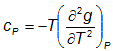is correct.

solution video  (large), (medium), (small)

P02.16        Heat Duty for Isobaric and Isochoric Heating of a Gas Using a High Precision Equation of State

A vessel is filled with nitrogen at J1 = 20°C and P1 = 1 bar. With the help of a high precision equation of state, calculate the duty
a) if the drum is heated up isobarically to J2 = 100°C.
b) if the drum is heated up isochorically to J2 = 100°C.
Interpret the results.Mathcad (2001) – Solution as XPS

P02.17        Relationship to Calculate the Entropy from the Derivative of the Gibbs Energy with Temperature at Constant Pressure
Show that the relationship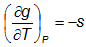is correct.

solution video  (large), (medium), (small)

P02.18        Vapor Pressure of Acetone from 7 Different EOS

Calculate the vapor pressure of acetone at T1 = 260 K, T2 = 360 K and T3 = 450 K using
a) the vapor pressure equation listed in Appendix A
b) a high-precision equation of state
c) the Peng-Robinson equation of state
d) the Redlich-Kwong-Soave equation of state
e) the Redlich-Kwong equation of state
f) the PSRK equation of state
g) the VTPR equation of state
What are the conclusions of the results ?
(All required parameters can be found in the appendix.)

(planned for June 2012)
Mathcad (2001) – Solution as XPS

P02.19        Density of Liquid Methanol from 7 Different EOS

Calculate the saturated density of liquid methanol at T1 = 300 K and T2 = 430 K using
a) the density equation listed in Appendix A
b) a high-precision equation of state
c) the Peng-Robinson equation of state
d) the Redlich-Kwong-Soave equation of state
e) the Redlich-Kwong equation of state
f) the PSRK equation of state
g) the VTPR equation of state
What are the conclusions of the results ?
(All required parameters can be found in the appendix.)

Mathcad (2001) – Solution (zip)      (planned for June 2012
)
Mathcad (2001) – Solution as XPS

P02.20        Compressibility Factor of Gaseous Propylene Via Tsonopoulos, Peng-Robinson and a High Precision Equation of State

Calculate the compressibility factor z of gaseous propylene at P1 = 2 bar and P2 = 10 bar at T = 293.15 K, using the Tsonopoulos and the Peng-Robinson equations of state. Check the results with a high-precision equation of state. All required parameters can be found in the appendix.

will be available soon
Mathcad (2001) – Solution as XPS

P02.21        Required Input Parameters for the Calculation of Saturated Vapor Enthalpy

Make a list of all the input parameters necessary for the calculation of the enthalpy of the saturated vapor of a pure substance if

a) the Peng-Robinson equation of state
b) the vapor pressure equation in combination with the Peng-Robinson equation of state
c) the VTPR equation of state

is used. The vapor pressure itself shall not be an input parameter.

P02.22        Saturated Vapor Fugacity, Vapor and Liquid Volumes and Heat of Vaporization Using Soave-Redlich-Kwong

Calculate
a) the fugacity fs at the saturation state
b) the molar volumes vL and vV in the saturation state
c) the enthalpy of vaporization
for n-butane, benzene and water at J1=30°C, J2=80°C and J3=130°C using the Soave-Redlich-Kwong equation of state. All required parameters can be found in the Appendix.In Appendix A, the Standard Gibbs energy of formation at J=25°C and P = 1 atm is reported to be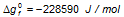. Estimate the Gibbs energy of formation for the liquid phase at J=25°C using the vapor pressure equation given in Appendix A.